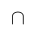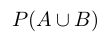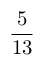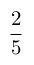Select Page

# CBSE Maths 12 Science Differential Equations MCQ Solutions in English

CBSE Maths 12 Science Differential Equations MCQ Solutions in English to enable students to get Solutions in a narrative video format for the specific question.

Expert Teacher provides CBSE Maths 12 Science Differential Equations MCQ Solutions through Video Solutions in English language. This video solution will be useful for students to understand how to write an answer in exam in order to score more marks. This teacher uses a narrative style for a question from Differential Equations not only to explain the proper method of answering question, but deriving right answer too.

Please find the question below and view the Solution in a narrative video format.

Question:

Solution Video in English:

You can select video Solutions from other languages also. Please check Solutions in ( Hindi )

## Similar Questions from CBSE, 12th Science, Maths, Differential Equations

Question 1 : Find the sum of the order and the degree of the following differential equation:(View Answer Video)

Question 2 : Form the differential equation of the family of circles in the second quadrant and touching the co-ordinate axes. (View Answer Video)

Question 3 : Find the solution of the differential equation(View Answer Video)

Question 4 : Write the degree of the differential equation(View Answer Video)

Question 5 : Find the particular solution of the differential equationgiven that y = 1 when x = 0.  (View Answer Video)

### Probability

Question 1 : Compute P(A/B) if P(B) =0.5 and P(AB)=0.32. (View Answer Video)

Question 2 : A bag A contains 4 black and 6 red balls and bag B 7 black and 3 red balls. A die is thrown. If 1 or 2 appears on it, then bag A is chosen, otherwise bag B. It two balls are drawn at random (without replacement) from the selected bag, find the probability of one of them being red and another black.        (View Answer Video)

Question 3 :  Four cards are drawn successively with replacement from a well shuffled deck of 52 cards. What is the probability that:
All the four cards are spades?

Question 4 : Evaluate, if 2P(A)=P(B)=and=. (View Answer Video)

Question 5 : A coin is biased so that the heads is 2 times as likely to occur as tail. If the coin is tossed twice, find the probability distribution of number of tails. Hence find the mean of the distribution.  (View Answer Video)

### Matrices

Question 1 :  Find the value of t, if(View Answer Video)

Question 2 : Using elementary transformation, find the inverse of the matrix. (View Answer Video)

Question 3 : Find the value of Y, ifand(View Answer Video)

Question 4 : If,find the value of x. (View Answer Video)

Question 5 : Find the value of Y, ifand. (View Answer Video)

### Inverse Trigonometric Functions

Question 1 : IfFind the values of x. (View Answer Video)

Question 2 :is equal to : (View Answer Video)

Question 3 : Evaluate :(View Answer Video)

Question 4 : Ifthen find the value of x. (View Answer Video)

Question 5 : Find the principal value of sin-1. (View Answer Video)